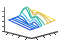# ribbon

•## Syntax

```ribbon(Y) ribbon(X,Y) ribbon(X,Y,width) ribbon(axes_handle,...) h = ribbon(...) ```

## Description

`ribbon(Y)` plots the columns of `Y` as three-dimensional ribbons of uniform width using `X = 1:size(Y,1)`. Ribbons advance along the x-axis centered on tick marks at unit intervals, three-quarters of a unit in width. Ribbon maps values in `X` to colors in colormap linearly. To change ribbon colors in the graph, change the `colormap`.

`ribbon(X,Y)` plots three dimensional ribbons for data in `Y`, centered at locations specified in `X`. `X` and `Y` are vectors or matrices of the same size. Additionally, `X` can be a row or a column vector, and `Y` a matrix with `length(X)` rows. When `Y` is a matrix, `ribbon` plots each column in `Y` as a ribbon at the corresponding `X` location.

`ribbon(X,Y,width)` specifies the width of the ribbons. The default is `0.75`. If `width = 1`, the ribbons touch, leaving no space between them when viewed down the z-axis. If ```width > 1```, ribbons overlap and can intersect.

`ribbon(axes_handle,...)` plots into the axes with handle `axes_handle` instead of the current axes (`gca`).

`h = ribbon(...) ` returns a vector of handles to surface graphics objects. `ribbon` returns one handle per strip.

## Examples

collapse all

Create a ribbon plot of the `peaks` function.

```[x,y] = meshgrid(-3:.5:3,-3:.1:3); z = peaks(x,y); figure ribbon(y,z)```## Version History

Introduced before R2006a